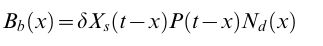# Solving delayed differential equations with Julia

Dear all,
I would like to solve some delayed differential equations that look like thiswhere t is a fix time and x is a variable time point.
I understand that there are specific packages that do this analysis, one being dde23 for matlab. I found this one for Julia. Are there other packages? And some introductory manual?
Thank you

I recommend starting with the tutorial: http://docs.juliadiffeq.org/latest/tutorials/dde_example.html

2 Likes

Thank you, I did but I can’t understand the logic behind. For instance, in the manual you cite – in relation to ordinary differential equations – they report that:

du/dt = f(u, p, t)
u(t) = u₀exp(αt)


so, how could it be that one can simply create an object
f(u,p,t) = 1.01*u
and simp[ly solve it with

u0=1/2
tspan = (0.0,1.0)
prob = ODEProblem(f,u0,tspan)


And this is about simple ODE, let alone more complex stuff. But I am not sure if this is the right forum to discuss such issues.
Thank you anyway.

Sorry, but I have difficulties understanding your equation to begin with.

It appears there is variable (time-dependent but not state-dependent) delay x (this may be confusing, as it is more common to use the symbols \tau or r for the delay) but I don’t understand what is the unknown, nor do I see a time-derivative anywhere. It looks like before you program anything, you may want to first write your equation such that it clearly has the form of a DDE with time-dependent delay.

(It is also important to understand that DDEs are quite unlike ODEs conceptually, in the sense that they require a piece of function (as opposed to an n-tuple of numbers) as the initial condition, but perhaps you knew that already.)

Thank you, I did but I can’t understand the logic behind. For instance, in the manual you cite – in relation to ordinary differential equations – they report that:

du/dt = f(u, p, t)
u(t) = u₀exp(αt)


so, how could it be that one can simply create an object
f(u,p,t) = 1.01*u
and simp[ly solve it with
u0=1/2
tspan = (0.0,1.0)
prob = ODEProblem(f,u0,tspan)

Well, is more an assertion: There is lot under the hood in this package. I am slowly studying how to use. My problem was more related with the lorenzian equations thus I am trying to work at that level. Thank you

.t-x turns out was the value of these equations at that time point, not the difference in time as I though, which implies I need the solver to identify those values… starting point is the least of the problems.